# SAT Math Multiple Choice Question 946: Answer and Explanation

### Test Information

Question: 946

14. Which of the following is a solution to the equation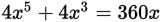? (Note: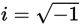)

• A. -10
• B.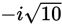• C. 10i
• D.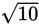Explanation:

B

Difficulty: Medium

Category: Additional Topics in Math / Imaginary Numbers

Strategic Advice: Plugging each choice into the equation will take far too long. Instead, factor the trinomial to find the solutions. Because this question does not allow the use of a calculator, look for ways to simplify the equation first, and then try obvious factors.

Getting to the Answer: Set the equation equal to 0, and then factor 4x out of the trinomial to get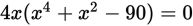. Factoring the new trinomial inside the parentheses (by finding the two factors of -90 that add up to +1) gives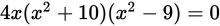. Set each factor equal to 0 and solve for x: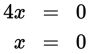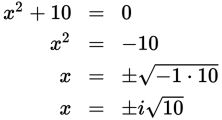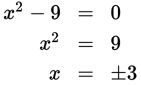The solutions are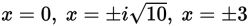. (B)is the only choice that contains one of the solutions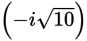.